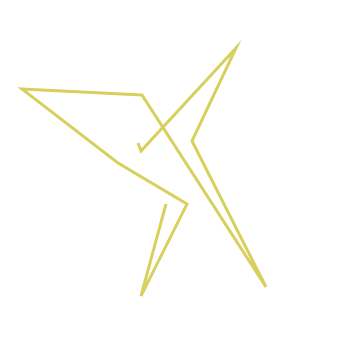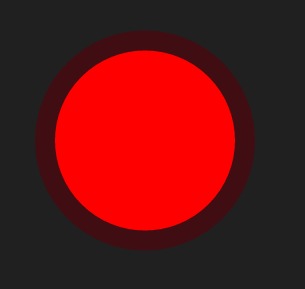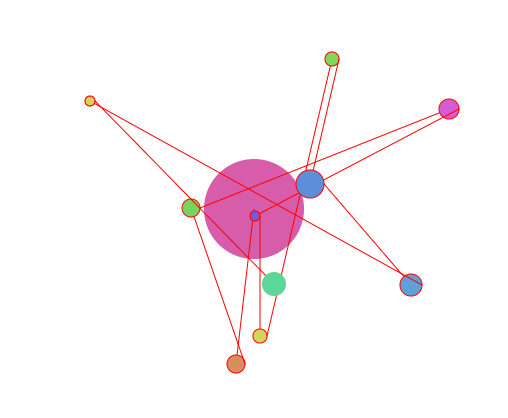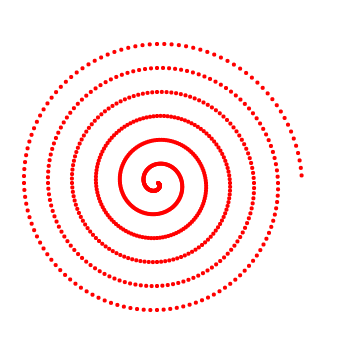## Canvas - Lines, Circles and Spirals

It’s time to branch beyond rectangles for our drawing elements. To draw a line between two points with the canvas api, using the context object:

``ctx.strokeStyle = "red";ctx.lineWidth = 5;ctx.beginPath();ctx.moveTo(0, 0);ctx.lineTo(100, 100);ctx.stroke();``

beginPath starts recording a path. Like Illustrator and some other drawing applications a path is just a set of points it doesn’t become an actual line or shape until a stroke or fill is applied. The canvas can have only one path active at a time.

lineTo(x,y) draws a path from wherever the last point was positioned on the current path to the point directed by x,y in lineTo. This initial point can be set by moveTo(x,y) or it can be wherever lineTo left off in the last call. For example:

``ctx.beginPath();ctx.moveTo(canvas.width / 2, canvas.height / 2);var color = randomInt(0, 360);ctx.strokeStyle = "hsl(" + color + ",60%,60%";for (var i = 0; i < 10; i++) {  ctx.lineTo(    randomInt(50, canvas.width - 50),    randomInt(50, canvas.height - 50)  );}ctx.stroke();``

We had to start the path with a call to moveTo(x,y) but after that we just piggybacked on wherever lineTo(x,y) left the last end point to begin the next line.## Circles

Rectangle is actually the only shape canvas knows how to draw. Everything else must be built from paths. A circle is created using the arc function and telling it the starting angle (in radians) is 0 and the ending is 2*PI:

``ctx.beginPath();ctx.arc(canvas.width / 2, canvas.height / 2, 100, 0, 2 * Math.PI, false);ctx.fillStyle = "red";ctx.fill();ctx.lineWidth = 20;ctx.strokeStyle = "#400d12";ctx.stroke();``Combining what we’ve covered so far:

``function draw() {  ctx.strokeStyle = "red";  ctx.lineWidth = 1;  drawCircle(canvas.width / 2, canvas.height / 2, 50);  ctx.beginPath();  ctx.moveTo(canvas.width / 2, canvas.height / 2);  for (var i = 0; i < 10; i++) {    var x = randomInt(50, canvas.width - 50);    var y = randomInt(50, canvas.height - 50);    ctx.lineTo(x, y);    ctx.stroke();    drawCircle(x, y, randomInt(5, 15));  }}function drawCircle(x, y, r) {  var c = randomInt(0, 360);  ctx.beginPath();  ctx.arc(x, y, r, 0, 2 * Math.PI, false);  ctx.fillStyle = "hsl(" + c + ",60%,60%";  ctx.fill();}``## Spirals

To draw a spiral we just need to draw a circle with a radius that either grows or shrinks.

``var radius = 1.0;ctx.translate(canvas.width / 2, canvas.height / 2);for (var deg = 0; deg < 360 * 6; deg += 3) {  var angle = (deg * Math.PI) / 180;  var x = Math.cos(angle) * radius;  var y = Math.sin(angle) * radius;  drawCircle(x, y, 2);  radius = radius + 0.2;}``Switching the formula in the line above from:

``var angle = (deg * Math.PI) / 180;``

to:

``var angle = (deg * 180) / Math.PI;``

## Textures

Besides colors, a fillStyle for a stroke or solid fill can also be a pattern or a gradient. Patterns are loaded from images and have an option for how they are tiled across a fill via the repeat parameter:

``// example from MDNvar img = new Image();img.src = "pattern.png";img.onload = function () {  var pattern = ctx.createPattern(img, "repeat");  ctx.fillStyle = pattern;  ctx.fillRect(0, 0, 400, 400);};``

Combining patterns with spirals was the focus of the next experiment

See the Pen Triangle Spirals by Kentskyo (@kentskyo) on CodePen.

Previous Canvas - Transforms Next Canvas - Triangles